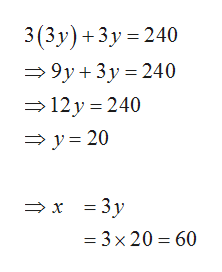# Suppose n(X)=240n(X)=240 and XX is partitioned into six subsets X1X1, X2X2, X3X3, X4X4, X5X5, and X6X6. All assume that n(X1)=n(X2)=n(X3)n(X1)=n(X2)=n(X3) and n(X4)=n(X5)=n(X6)n(X4)=n(X5)=n(X6). If there are 3 times more elements in X1 as there are in X5, find the following values: n(X1)n(X2)n(X3)n(X4)n(X5)n(X6)

Question
Suppose n(X)=240n(X)=240 and XX is partitioned into six subsets X1X1, X2X2, X3X3, X4X4, X5X5, and X6X6. All assume that n(X1)=n(X2)=n(X3)n(X1)=n(X2)=n(X3) and n(X4)=n(X5)=n(X6)n(X4)=n(X5)=n(X6). If there are 3 times more elements in X1 as there are in X5, find the following values:

n(X1)
n(X2)
n(X3)
n(X4)
n(X5)
n(X6)
check_circleExpert Solution
Step 1

The given details are

n(X) = 240

n(X1) = n(X2) = n(X3) = x

n(X4) = n(X5) = n(X6) = y

n(X1) = 3n(X5), (i.e.,) x = 3y

Observe the following from the above details.

Step 2

Obtain the value of x and y ...help_outlineImage Transcriptionclose3(3y) 3y 240 9y 3y 240 12 y 240 y20 x 3y - 3 x 20 60 fullscreen

### Want to see the full answer?

See Solution

#### Want to see this answer and more?

Solutions are written by subject experts who are available 24/7. Questions are typically answered within 1 hour*

See Solution
*Response times may vary by subject and question
Tagged in

### Math